# Puzzle Walkthrough For Professor Layton And The Unwound Future – The Newest Of The Professor Layton Games

## Professor Layton: Puzzle Solutions For Puzzles 103-110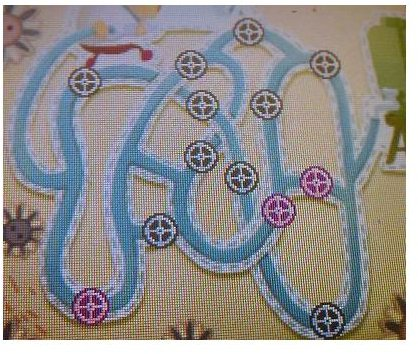Puzzle #103 – Pool Problem:

Goal: Replacing only three of the faulty valves in the image, get the water to flow through the hose.

Solution: The three valves you need to replace are shown in the image to the right.

Puzzle #104 – The Cake Gobbler:

Goal: Identify which of the people (A, B, C, or D) is lying about not eating the cake.

Solution: This puzzle is incredibly mean. Notice that you circle your answer instead of tapping a letter, like in most multiple choice puzzles. That’s actually because all four people are telling the truth, and the accuser is the one lying. Circle the fifth person on screen that has no letter above his head for your answer.

Puzzle #105 – The Fingerprint:

Goal: Choose which fingerprint (A, B, C, or D) matches the image underneath the magnifying glass.

Solution: Remember that a fingerprint left behind on something would be a mirror image of the print looking down on the actual fingertip. The solution is A.

Puzzle #106 – Four Stamps:

Goal: Determine which of the four stamps you would have to use to create a valid equation in the space given using the fewest number of stamps possible.

Solution: As usual with puzzles like this, your task is to create a number not given to you with the numbers you have, but for once the solution isn’t to flip a 6 upside-down to get a 9. The valid equation here is 3+5=8, or 5+3=8, using the 3 stamp twice (once backwards) to form an 8. The stamps you need are 3 and 5.

Puzzle #107 – Chicken Race:

Goal: Just like in photo finish earlier, you need to use the torn apart and shuffled pieces of a photo taken of a race to determine the placements, and then you need to identify 7th place to solve the puzzle.

Solution: Again, the challenge here comes with being able to put the pieces back together in your head and keep them straight while you do. If you manage it, you’ll find that E is in 7th place.

Puzzle #108 – Dogs And Cats:

Goal: Determine the smallest value possible to make the very strange equation true.

Solution: If you plug some values into the example equations you’re given, the pattern you’ll find is that odd numbers are represented as “dog” and even numbers are represented as “cat.” Thus, to complete the equation “1 + ? = dog dog dog” you simply need to plug in the smallest value that results in three consecutive odd numbers when added to 1. The solution is 110.

Puzzle #109 – Three Blocks:

Goal: Rotate the maze clockwise and counter-clockwise to get the red, blue, and yellow blocks to fall into their respective spaces.

Solution: Rotate counter-clockwise twice, then clockwise five times, the counter-clockwise another five times, and finally, clockwise three times.

Puzzle #110 – A Zero Sum Game:

Goal: Change all four tiles to zeroes, using the four buttons.

Solution: It doesn’t tell you what changes each button makes, but if you play around with it a bit, you’ll find that button A adds five to its column, and button B adds 3. Button C adds 7 to its row, and button D adds 4. Using this knowledge, the solution is as follows: Press A once, followed by B nine times, C seven times, and D four times.

## Puzzles 111-115

Puzzle #111 – Follow The Code:

Goal: Following the squares in the order given to determine which square (A, B, C, or D) will unlock the door.

Solution: The only square you can reach following the instructions is A. The solution to this puzzle is A.

Puzzle #112 – Beams And Ladders:

Goal: Determine which order you will cross the three highlighted ladders to travel across the entire maze without ever crossing your own path.

Solution: There are a few possible paths, but they all use the three ladders in the same order. The one in the top middle space is number 1, the one on the right is number 2, and the one in the bottom center space is third.

Puzzle #113 – One Step At A Time:

Goal: Using the conditions that each square can only go up or down by one number, chart the path from beginning to end of the maze.

Solution: From the start, go down to the 2 below you, then down one to the 3. From there, move one right to the 4, then down to 3, down to 2, down to 3, right to 4, right to 5, right to 6, up to 5, up to 4, right to 3, down to 2, and finally, down to 1 and the exit.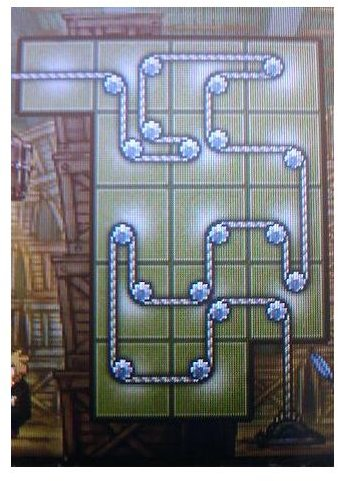Puzzle #114 – Roped In:

Goal: Re-arrange the tiles to connect the rope from your scissors to the wooden crate above the goons’ head.

Solution: With the two lines of ropes, this one can be a tricky one to visualize any patterns in. The easiest way to solve it is probably to just start shuffling tiles around at random until it starts to come together. The correct arrangement that you should come to if you do is shown in the image to the right.

## Puzzles 115-119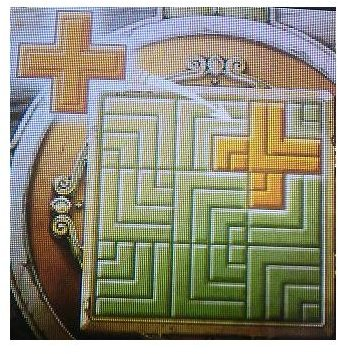Puzzle #115 – Hidden In Plain Sight:

Goal: Find the plus sign hidden in the pattern of lines that makes up the grid.

Solution: The lines are drawn in specific patterns designed solely to make this hard to see, but there is a plus sign you can trace around in the top right corner, as shown to the right.

Puzzle #116 – Which Way Is Up?

Goal: Determine which side of the map (A,B,C, or D) is North given the directions.

Solution: You need to follow the direction given on each square and find which orientation of the map allows you to travel through every square by doing so. Using C as North is the only possible solution.

Puzzle #117 – Where’s The Arrow?

Goal: Find the tile with the hidden treasure underneath it by finding the hidden arrow in the room that points to it.

Solution: This isn’t the best puzzle, because there’s more than a couple arrow-shaped objects in the room. The monkey’s nose, however, in the painting to the left, is the most arrow-shaped, and the tile underneath it that it points to is the solution.

Puzzle #118 – Scrutinized Screws:

Goal: Choose the screw needed (A, B, C, D, E, or F) to complete the restoration of the Laytonmobile

Solution: You only have a flathead screwdriver, so you can rule out B and F, and beyond that it’s a matter of being able to visualize the threads on the screw versus the threads in the hole and determine which one will screw in. The solution is D.

Puzzle #119 – Swap To Unlock:

Goal: Move around and rotate the 6 tiles so that all adjacent gems, horizontally or vertically, are the same.

Solution: Place tile F in the top left corner and rotate it 90 degrees clockwise. Place C underneath that, and rotate it 90 degrees clockwise as well. Move D to the bottom left; it doesn’t need to be rotated at all. E doesn’t need to be rotated either, and can go in the top right. B should be underneath that, rotated 90 degrees clockwise. Finally, A goes in the bottom right without needing to be rotated. Submit that arrangement and you’ll have this puzzle solved.

## Puzzles 120-123

Puzzle #120 – A Cryptic Combo:

Goal: Determine which numerical tiles on the panel the clues are trying to tell you to highlight.

Solution: “Open with a two, but leave the four” has absolutely nothing to do with the numbers shown on the tile. They want you to draw a number 2 out of the tiles you press, leaving 4 unlit. Click on all three buttons on the top row, the 6 in the second row, all three buttons in the third row down, the 10 in the fourth row, and all three buttons on the bottom row. Submit that to solve.

Puzzle #121 – Arrow Flow:

Goal: Find the starting tile that allows you to move through every tile on the maze, following the arrows.

Solution: Since each arrow points to the next arrow you need to move to, the way to solve this is to simply find the one tile that no other tiles point to, and that’s your start. One square up and one square to the left of the bottom right corner is where you’ll find that tile.

Puzzle #122 – Password Pieces:

Goal: Determine the numerical password on the 10 puzzle pieces by putting them back together.

Solution: This one is pretty straight forward. Find out where the pieces go and then read the number off the image once you’ve got them in place. The password is 081127.

Puzzle #123 – Cat’s-Eye View:

Goal: Determine the maximum number of stones that can be placed in the grid allowing for no four stones to form a square or rectangle horizontally or vertically anywhere on the grid.

Solution: There are a couple of ways to arrange them, but no matter how you do, the maximum number of stones you can place on the grid without forming a square or rectangle is 9.

## Puzzles 124-126

Puzzle #124 – The Final Tile:

Goal: Using the sequence given to you, determine which tile is going to fall last.

Solution: The key hint is that neighboring tiles are set to fall away one at a time. That means that anywhere you come to where there are 2 adjacent tiles that are next in sequence is an indication that you’re following the wrong sequence, which you should be able to use to determine the first tile. From there, you simply have to follow the path down to the last tile, which is the spade on square up and one square to the right from the bottom left tile.

Puzzle #125 – Connect The Bots:

Goal: Determine which room is the generator room by finding the one room the security bots don’t go past.

Solution: By drawing out the loops each bot follows, you’ll find there’s only one room they both avoid. Click on the room that is two squares up and two squares to the left from the bottom right corner to solve.

Puzzle #126 – Ten Step Solution:

Goal: Swap blocks A and B to opposite sides of the slide puzzle, but do so in ten steps or less.Solution: The solution is provided here, step-by-step, and as always with slide puzzles, the pieces are labeled with letters in the image to the right. Also, when doing this puzzle, drag each piece as many squares as it needs to move without lifting the stylus so it counts as a single move.

• Slide the second bar from the top (piece C) one square left.
• Then, move the A block (A) down two spaces, right one, and down one.
• Next, slide C two squares to the right.
• Drag the second bar from the bottom (piece D) left one square and then up one square.
• Slide the B block (B) up two squares and left one square.
• Slide A left two squares and down two, into its space.
• Then drag B three spaces to the right.
• Next, move D down one square.
• Drag C left two squares.
• Finally, slide B one square to the left and three squares up into its space as your tenth and final move.

## Puzzles 127-132

Puzzle #127 – A Real Heart Swapper:

Goal: In just one move, swap two tiles so that the wires running from the generator connect to the pocket watch instead of the heart monitor.

Solution: The wires are pretty tangled and the path can be hard to follow, but if you swap the second and fourth tiles in the center row, you’ll solve this puzzle.

Puzzle #128 – Reverse Rotation:

Goal: Reverse the direction of gear B by placing all or some of the extra cogs provided into the machine.

Solution: As you know from earlier gear-related puzzles, an even number of cogs between the gears will cause them to spin in opposite directions. To achieve this, you’ll need to use pieces B and C. A is unnecessary. Remove A and place C in its place. Then, rotate B 180 degrees and place it on the top left of the center cog. The puzzle will now be solved.

Puzzle #129 – Block Blockade:

Goal: Use the five bombs to blow up just the right pieces to create a flat surface for the Laytonmobile to drive across.

Solution: Gravity is acting on these, so every block you blow up will cause the block above it to fall. With that in mind, place your bombs as follows, from left to right. The yellow T-shaped piece, the orange L-shaped piece above it, the yellow Z-shaped pieced to the right of that, the orange T-shaped piece to the right of that, and finally, the blue L-shaped piece in the bottom right hand corner. Press the button to detonate and the puzzle will be complete.

Puzzle #130 – The Final Puzzle:

Goal: Luke has made an L-shaped wax stamp to seal his letters, and you need to determine which of the four examples (A, B, C, or D) matches his stamp.

Solution: All you need to do is match the image to the example and remember to flip it because the imprint from the stamp is going to be a mirror image of the stamp itself. The solution is C.

Puzzle #131 – A Pricey Pen:

Goal: Determine the profit the woman made reselling her expensive purchase.

Solution: The \$3000 pen was bought at a 10% discount, which is 2700, and sold for 10% more than she paid, which is 2970, so she made a profit of \$270.

Puzzle #132 – Calendar Collage:

Goal: Determine which month is represented by the page the arrow is pointing to in the strange calendar collage.

Solution: It’s easy to tell that each consecutive month is going to be on top of the month before it, so you just need to determine how many pages are above and below the one in question, but it can be hard to follow which pages are above each other with the way they’re laid out. There are actually no pages below the one indicated, however, making the answer January, or 1.

## Puzzle 133-135

Puzzle #133 – The Two Necklaces:

Goal: Switch the positions of the red and blue necklaces.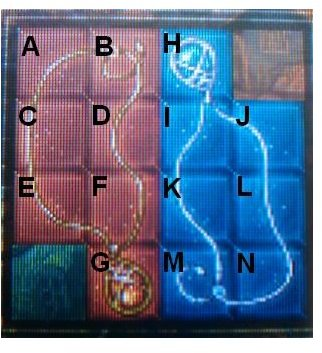Solution: Unlike most slide puzzles, this has two empty spots at either side, which makes it go a bit smoother. However, re-assembling the two pieces is more complex than the game’s usual task of moving one piece to an exit. In any case, the step-by-step solution follows here, and pieces are labeled in the image to the right.

• Slide the bottom left red block (piece E) down one square.
• Next, drag the red block beside it (piece F) one square to the left.
• Then, move the bottom right red block (piece G) up one space.
• Slide the bottom left blue piece (piece M) one square to the left.
• Move the blue square beside that (piece N) one square left.
• Slide the blue block above that (piece L) down one square.
• Next, drag the blue block beside that (piece K) one space right.
• Drag G one square to the right.
• Now move F one space to the right.
• Next, move E one square up.
• Slide M one square left.
• Then, slide N one square left.
• Drag G down one space.
• Slide the top blue block (piece H) one square to the right.
• Slide the blue block below that (piece I) one space up.
• Then, move K one square left.
• Slide L up one square.
• Drag G right one space.
• Drag K down one square.
• Then move F right one square and up one square.
• Drag K up one square and left one square.
• Now, slide F down two squares.
• Drag the red piece one below the top right (piece D) to the right one space.
• Next, slide the red block beside it (piece C) to the right one space.
• Move E up one square.
• Move K left one space.
• Now, slide C down one square.
• Next move D down one square.
• Now, drag E two spaces to the right.
• Move C up one square and left one square.
• Then drag D up one space and to the left one space.
• Now move L two spaces left.
• Drag F up one square and to the right one square.
• Now drag E down two squares.
• Move D down one square and right one square.
• Then slide I down one square and one to the left.
• Next, move H down one and one to the left.
• Now, drag the top right red block (piece B) two squares to the right.
• After that, slide the top left red block (piece A) two squares to the right as well.
• Move C up one square and to the right one square.
• Slide I to the left one square.
• Then, slide H to the left one square as well.
• Drag A down one square.
• Now, move C to the right one square.
• Move H up one square and to the left one square.
• Move A up one space and to the left one space.
• Now, drag the last blue block (piece J) two squares to the left.
• Move D up one square and right one square.
• Then, drag C down one square.
• Now, move E up one square.
• Finally, slide A to the right one square to complete the puzzle.

Puzzle #134 – A Question Of Taste:

Goal: Determine which of the fine suits (A, B, C, or D) appeals most to Professor Layton.

Solution: Since you’re given nothing extra to go on, you’ll need to rely on what you know, which is that the Professor likes puzzles, and C is being hung on a clothes hanger shaped like a question mark. The solution is C.

Puzzle #135 – Four Jams:

Goal: Use the scales to find the weight of the four jams.

Solution: By looking at which jams are repeated in different pairs and comparing step-by-step, you can find that Blue is the heaviest, followed by Yellow, then Green, and then Red. Label them 1 through 4, lightest to heaviest, so in the order, red, green, yellow, blue.

## Puzzles 136-140

Puzzle #136 – Destination Station:

Goal: If the person knows which station a train passenger is getting off at, determine which of the four trains (A, B, C, or D) that passenger would have to be riding for him to know that.

Solution: Train D only has one station remaining on the line, which means that anyone would be able to “guess” which station its passengers were going to get off at. D is the solution.

Puzzle #137 – Pieces of Chocolate:

Goal: Determine how many pieces the chocolate bar can be broken into.

Solution: You’re given the dimensions of the bar and the dimensions the pieces need to be, so it would just be simple math if not for the small hole in the chocolate bar. Either way, you simply need to make it so that hole exists in only one of your pieces, thus wasting only one section of the chocolate, and allowing you to break off eight pieces. The solution is 8.

Puzzle # 138 – Quirky Clockwork:

Goal: Determine which day of the month shown (A, B, or C) could not appear on the clock’s dial.

Solution: All three are days that don’t exist, but B and C both make sense as days the dial would momentarily show as it was rotating between specific dates. For C to ever happen, there would have to be at least 47 days in the month. C is the solution.

Puzzle #139 – Bricks N’ Bullion: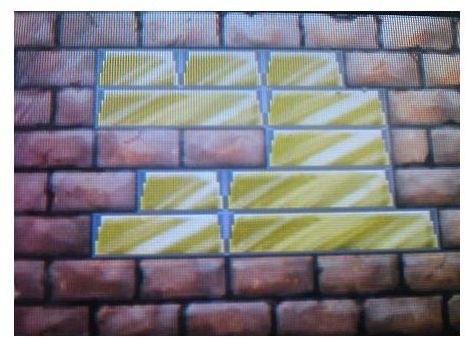Goal: Place the gold bars into the hole in the brick wall so that they fit perfectly and no piece is stacked on top of a piece smaller than it.

Solution: There are four sizes of gold bricks. The smallest, which are the size of actual bricks, and three incrementally larger sizes. We’ll call them small, medium, large, and extra large. First place a medium brick in the bottom left, and the extra large brick next to it. Place a small brick on top of the medium, and a large brick on top of the extra large. Place a medium brick on top of the large brick on the right side and another medium brick on top of that. Place the last large brick to the left on that on top of the two actual bricks. Now, line the entire top row with the three remaining small bricks. The solution is shown to the right.

Puzzle #140 – Ripple Effect:

Goal: Count the criss-crossing ripples in the pond to determine how many pebbles fell into it.

Solution: With all the lines crossing each other, it can be easy to mistake certain lines for a part of ripples they don’t belong to, so keep a close eye on the exact shape of the curves. There are 6 total sets of circles, so the answer is 6 pebbles.

## Puzzles 141-143

Puzzle #141 – A Roll Of The Die:

Goal: Match the shapes on the die to the path shown to determine what shape will appear in the last space.

Solution: The key to this is to not only drag the correct space into the final tile, but to make sure you rotate it into the correct orientation. The answer is an upside-down heart.

Puzzle #142 – The Marked Cup:

Goal: Using the knowledge that one cup is marked, choose which of the three cups (A, B, or C) has the coin underneath it after two cups switch places.

Solution: The cup with the coin underneath it is the one that didn’t move, and because of the mark on cup C, you know the only cup that didn’t move is B. Select B to solve the puzzle.

Puzzle #143 – Double Digits:

Goal: Cut the board into two identical shapes that also look like a number.

Solution: The shape you want to cut the boards into is the number 4. Start one square down from the top left and cut one space to the right, then three down, one to the right, three up, one to the right, three down, and finally, one more to the right. That will form two identical number 4 shapes.

## Puzzles 144-148

Puzzle #144 – Mr. 8:20:

Goal: Identify which of the five men (A, B, C, D, or E) has the nickname “Mr. 8:20”

Solution: They’re all wearing clothing that looks vaguely like it might be the answer, but people change their clothes, so a nickname wouldn’t come from that. However, if you envision the ends of E’s mustache as clock hands, they would be pointing to 8:20. E is the answer.

Puzzle #145 – Time Cards:

Goal: Determine how many times can be made on a 24-hour clock using the four number cards 0, 1, 2, and 6.

Solution: The key is to only form real answers, so no hours higher than 23, and no minutes higher than 59. The total number of possible combinations is 20.

Puzzle #146 – Birds On A Wire:

Goal: Based on the story, determine how many birds were originally perched on the wire.

Solution: Half always fly away, and one always comes back. For that pattern never to change, the “half” has to be referring to one. There are two birds, and half (one) flies away, then one comes back, returning the amount of birds to two. The original number of birds is 2.

Puzzle #147 – Paving The Garden:

Goal: Choose which single shape (A, B, C, D, or E) can be used to fill in the entire space completely without ever overlapping.

Solution: The piece that can be rotated to fill in the entire space without leaving any spaces empty or ever overlapping is E.

Puzzle #148 – Matchstick Math:

Goal: Identify the fewest number of matches you would need to move to make the equation shown become valid.

Solution: This one’s a little mean. You can actually form a valid equation by flipping the image upside down, without technically moving a single match. The solution is 0.

## Puzzles 149-153

Puzzle #149 – Strange Symbols:

Goal: Determine the number that shape C signifies.

Solution: It’s hard to see, but using the fact that A is 63 and B is 89, you can identify the pattern. The empty space around the symbols, when rotated 90 degrees to the right, spells out the numbers. And, following that pattern, shape C signifies the number 52.

Puzzle #150 – Half As Old:

Goal: Find the youngest sister’s age.

Solution: The language and the tenses here are pretty misleading. A year ago, the younger sister was half her older sister’s age. A year from now, the older sister will be double her younger sister’s current age, not the age she’ll be in a year. The only age for that to be possible is 9. The younger sister’s age is 9 years old.

Puzzle #151 – A Difference Of 39:

Goal: By changing a single tile on each side of the image showing the number 58, create two numbers with a difference of 39 when one is subtracted from the other and input what those two numbers will be.

Solution: By changing a single tile on each, you can create the numbers 98 and 59. 98-59=39. The solution is the two numbers 98 and 59.

Puzzle #152 – Cluttered Cans:

Goal: Re-arrange the cans so each stack is a stack of the same product, and do so in less than six moves.

Solution: The solution is as follows, step-by-step.

• Move the top two cans from the center stack to the left hand stack.
• Then move the top can from the right stack to the center.
• Next, lift the top four cans from the left stack to the center.
• Then, move the top can from the center to the left stack.
• Now, lift the top can from the right stack to the left stack.
• Finally, move the top two cans from the center to the right stack. The puzzle will now be solved.

Puzzle # 153 – An Extra Block:

Goal: Choose which one of the six smaller shapes is not used in the construction of the one large shape.

Solution: By rotating and flipping five of the six shapes, you can form the larger shape. The only piece not needed is the smallest of the 6 shapes. Circle the small piece composed of only 2 triangles to solve the puzzle.

## This post is part of the series: Professor Layton and the Unwound Future Puzzle Walkthrough

Stuck on a puzzle in the most recent entry in the Professor Layton series? These articles will walk you through the solution to every single puzzle in the game, including all of the available bonuses, and all of the weekly downloadable puzzles. Read on for all the answers you’ll need.